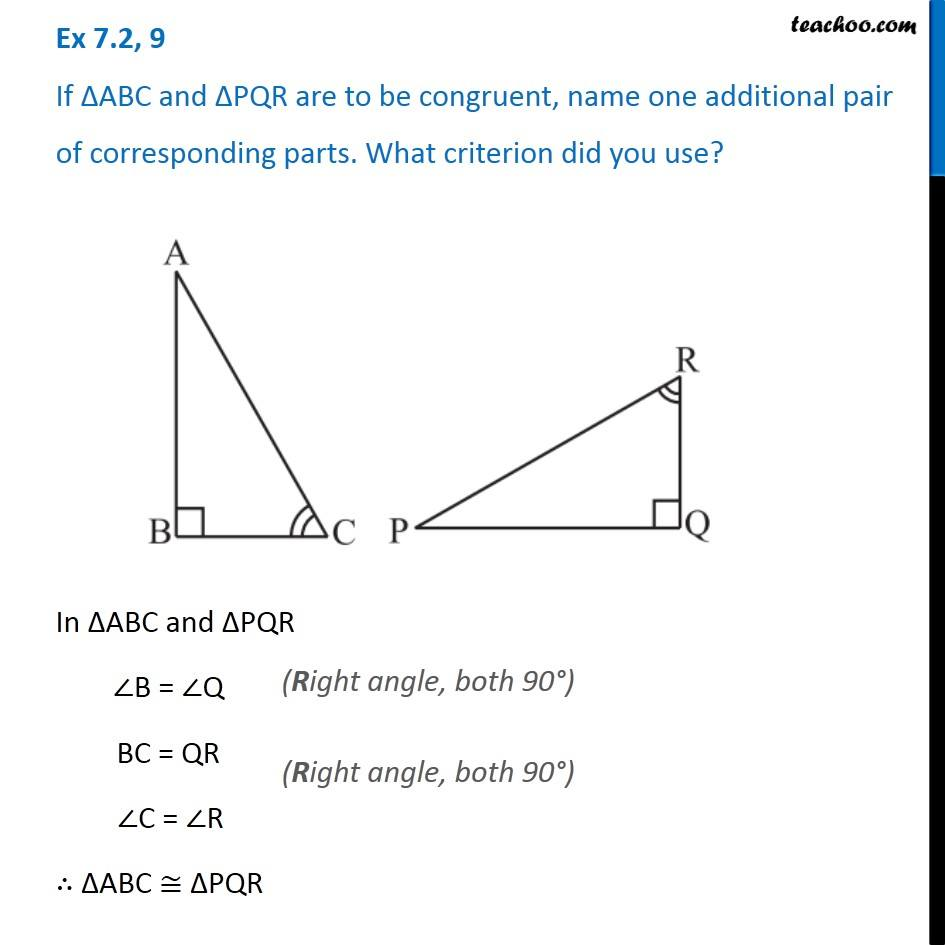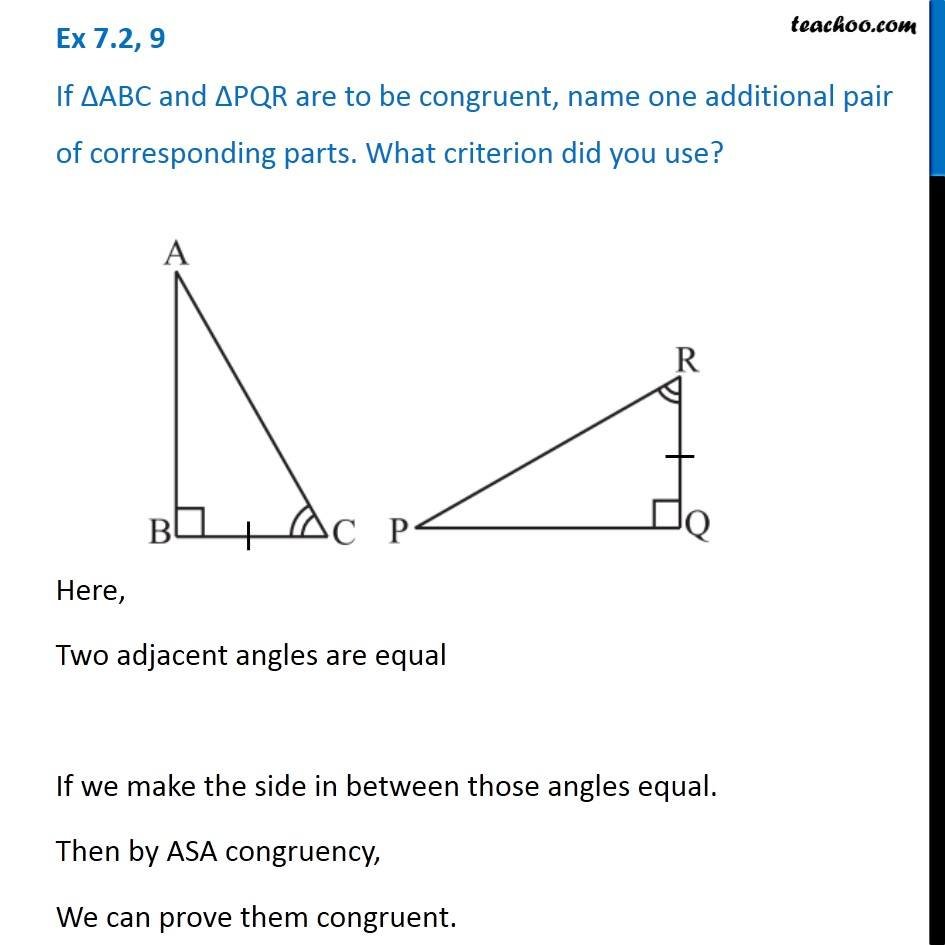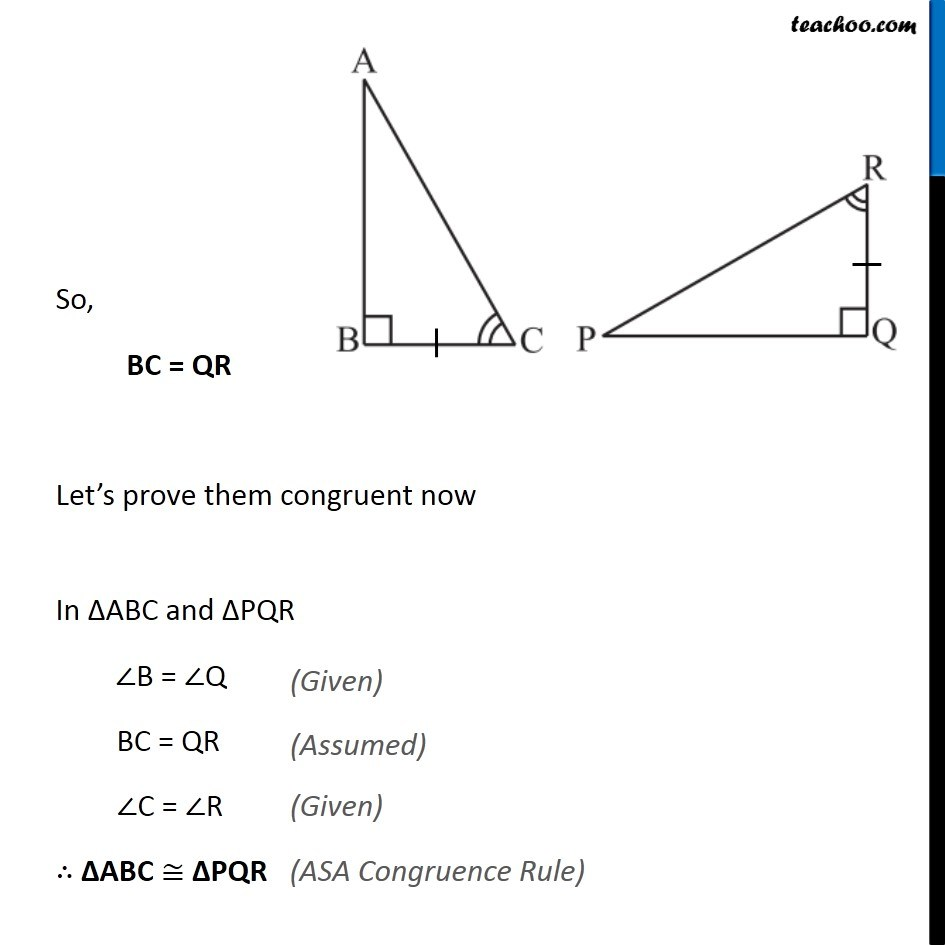Congruency - Different Criteria's

Congruence of Triangles
Serial order wiseLearn in your speed, with individual attention - Teachoo Maths 1-on-1 Class

### Transcript

Question 9 If ∆ABC and ∆PQR are to be congruent, name one additional pair of corresponding parts. What criterion did you use?In ∆ABC and ∆PQR ∠B = ∠Q BC = QR ∠C = ∠R ∴ ∆ABC ≅ ∆PQR Question 9 If ∆ABC and ∆PQR are to be congruent, name one additional pair of corresponding parts. What criterion did you use?Here, Two adjacent angles are equal If we make the side in between those angles equal. Then by ASA congruency, We can prove them congruent. So, BC = QR Let’s prove them congruent now In ∆ABC and ∆PQR ∠B = ∠Q BC = QR ∠C = ∠R ∴ ∆ABC ≅ ∆PQR